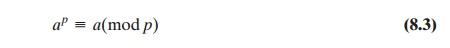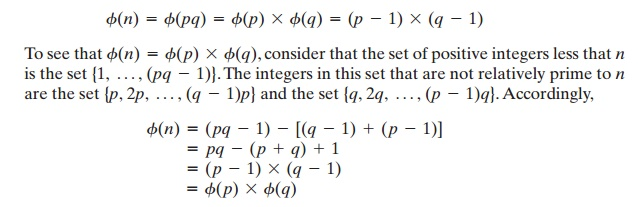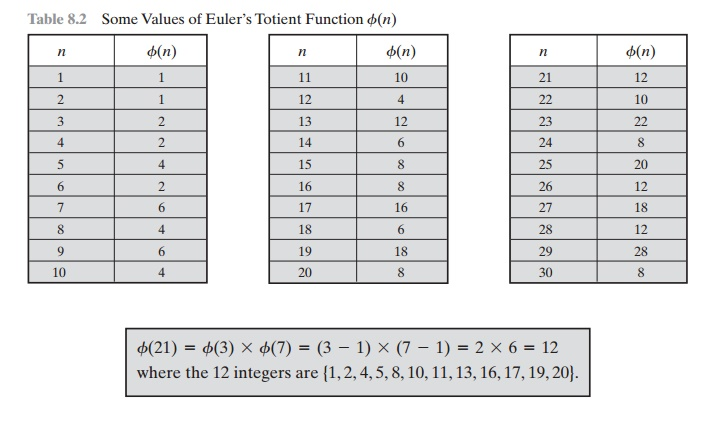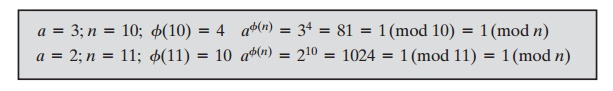Home | | Cryptography and Network Security | Fermat‚Äôs And Euler‚Äôs Theorems

# Fermat‚Äôs And Euler‚Äôs Theorems

Two theorems that play important roles in public-key cryptography are Fermat‚Äôs theorem and Euler‚Äôs theorem.

FERMAT‚ÄôS AND EULER‚ÄôS THEOREMS

Two theorems that play important roles in public-key cryptography are Fermat‚Äôs theorem and Euler‚Äôs theorem.

Fermat‚Äôs Theorem

Fermat‚Äôs theorem states the following: If is prime and is a positive integer not divisible by p, thenProof: Consider the set of positive integers less than p: {1, 2,  ......., p  -   1}  and multiply   each   element  by a,  modulo p,   to   get   the  set X = {a mod p,  2a mod p, ..... , (p - 1)a mod p}. None of the elements of X is equal to zero because p does not divide a. Furthermore, no two of the integers in X are equal. To see this, assume that ja == ka (mod p)), where 1 <= <= 1. Because is relatively prime5 to p, we can eliminate a from both sides of the equation [see Equation (4.3)] resulting in j === k (mod p). This last equality is impossible, because j and k are both positive integers less than p. Therefore, we know that the (p - 1) elements of X are all positive integers with no two elements equal. We can conclude the X consists of the set of integers {1, 2, ...., p - 1} in some order. Multiplying the numbers in both sets (p and X) and taking the result mod p yieldsWe can cancel the ((1)! term because it is relatively prime to [see Equation (4.5)]. This yields Equation (8.2), which completes the proof.An alternative form of Fermat‚Äôs theorem is also useful: If is prime and is a positive  integer, thenNote that the first form of the theorem [Equation (8.2)] requires that a be relatively prime to p, but this form does not.Euler‚Äôs Totient Function

Before presenting Euler‚Äôs theorem, we need to introduce an important quantity in number theory, referred to as Euler‚Äôs totient function, written œï(n), and defined as the number of positive integers less than n and relatively prime to n. By convention, œï(1)   =   1.

DETERMINE œï(37) AND œï(35).

Because 37 is prime, all of the positive integers from 1 through 36 are rela- tively prime to 37. Thus œï(37)   =   36.

To determine œï(35), we list all of the positive integers less than 35 that are rela- tively prime to it:

1, 2, 3, 4, 6, 8, 9, 11, 12, 13, 16, 17, 18

19, 22, 23, 24, 26, 27, 29, 31, 32, 33, 34

There are 24 numbers on the list, so œï(35)  =  24.

Table 8.2 lists the first 30 values of œï(n). The value œï(1) is without meaning but is defined to have the value 1.

It should be clear that, for a prime number p,

œï(p)  =  p  -  1

Now suppose that we have two prime numbers and with != q. Then we can show that, for pq,Euler‚Äôs Theorem

Euler‚Äôs theorem states that for every and that are relatively   prime:Proof:   Equation (8.4) is true if n is prime, because in that case, œï(n)  =  (n  -  1) and Fermat‚Äôs theorem holds. However, it also holds for any integer n. Recall that f(n) is the number of positive integers less than n that are relatively prime to n. Consider the set of such integers, labeled as

R  =  {x1x2,  ...... xœï(n)}

That  is,  each  element xis  a  unique  positive  integer  less  than  with gcd(xin)  1. Now multiply each element by a, modulo n:

S  =  {(ax1 mod n), (ax2 mod n),  ....., (axœï(n) mod n)}

The set is a permutationof R, by the following line of reasoning:

1.                                                                                  Because is relatively prime to and xis relatively prime to naxmust also be relatively prime to nThus, all the members of are integers that are less than and that are relatively prime to  n.

1. There are no duplicates in S. Refer to Equation (4.5). If axi mod n = axj mod n, then xi = xj.

Therefore,which completes the proof. This is the same line of reasoning applied to the proof of Fermat‚Äôs theorem.As is the case for Fermat‚Äôs theorem, an alternative form of the theorem is also useful:Again, similar to the case with Fermat‚Äôs theorem, the first form of Euler‚Äôs theorem [Equation (8.4)] requires that be relatively prime to n, but this form does   not.

Study Material, Lecturing Notes, Assignment, Reference, Wiki description explanation, brief detail
Cryptography and Network Security Principles and Practice : Asymmetric Ciphers : Introduction to Number Theory : Fermat‚Äôs And Euler‚Äôs Theorems |